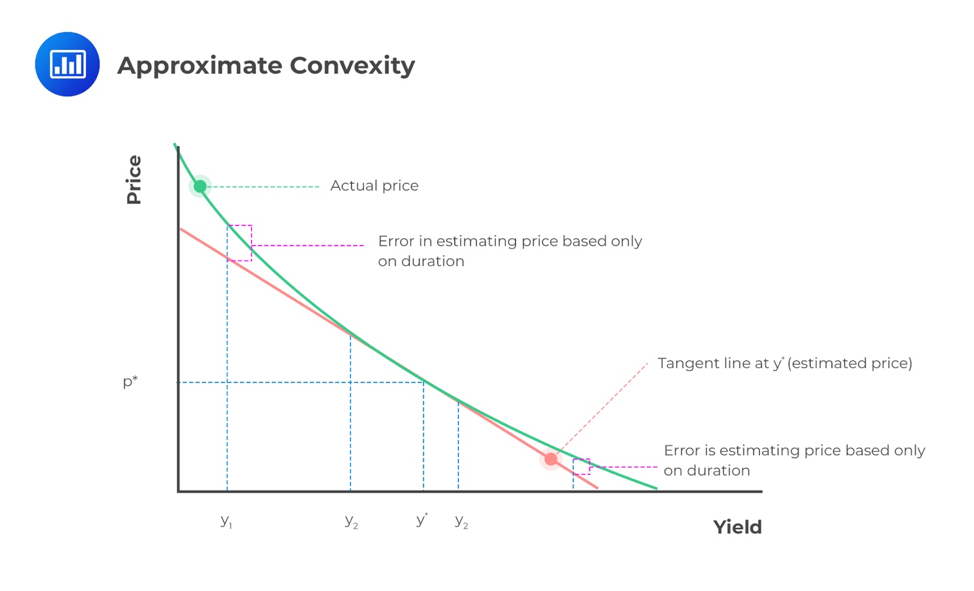# Calculate and Interpret Convexity

The Modified Duration provides an estimate of the percentage price change for a bond given a change in its yield-to-maturity. A secondary effect is measured by the convexity statistic.

## Approximate Convexity

The true relationship between the bond price and the yield-to-maturity (YTM) is a curved (convex) line. The duration (in particular, money duration) estimates the change in bond price along with the straight line that is tangent to the curved line. For small yield-to-maturity changes, there is little difference between the lines. However, as the change in YTM grows larger, the difference becomes significant.A convexity measure is used to improve the estimate of the percentage price change.

$$\%ΔPV^{FULL}≈(\text{-AnnModDur}×ΔYield)+(\frac{1}{2}×\text{AnnConvexity}×(ΔYield)^2)$$

The second bracketed expression is the convexity adjustment:

$$\text{Convexity effect} ≈ \frac{1}{2}×\text{AnnConvexity}×(ΔYield)^2$$

The convexity adjustment is the annual convexity statistic, AnnConvexity, times one-half, multiplied by the change in the yield-to-maturity squared. This amount adds to the linear estimate provided by the duration alone, which brings the adjusted estimate very close to the actual price on the curved line. The sign “≈” is used because the resulting adjusted price is just an estimate.

## Effective Convexity

The effective convexity of a bond is a curve convexity statistic that measures the secondary effect of a change in a benchmark yield curve. Note that for bonds with somewhat unpredictable cash flows, we use effective duration to measure interest rate risk. Similarly, we use the effective convexity to measure the change in price resulting from a change in the benchmark yield curve for securities with uncertain cash flows. In fact, effective convexity is a secondary curve convexity statistic that measures the secondary effect of a change in the benchmark yield curve.

$$\text{Effective convexity}=\frac{PV_{-}+PV_{+}-2PV_{0}}{(ΔCurve)^2PV_{0}}$$

Where:

PV = Price if yield curve declines by Yield (parallel shift)

PV+ = Price if yield curve  increases by Yield (parallel shift)

PV0 = Initial bond price

ΔCurve = Change in yield on the benchmark curve

The main difference between approximate and effective convexity is that approximate convexity is based on a yield to maturity change, whereas effective convexity is based on a benchmark yield change.

Here are a few quick facts about bonds with embedded options:

• when the benchmark yield is high, prices of callable and non-callable bonds change almost in tandem with interest rate changes;
• when the benchmark yield is low, the value of the call option (to the issuer) increases;
• putable bonds always have positive convexity;
• callable bonds exhibit negative convexity.

## Zero-coupon Bonds

For a zero-coupon bond, the exact convexity statistic in terms of periods is given by:

$$\text{Convexity}_{\text{zero-coupon bond}}=\frac{[N-\frac{t}{T}]×[N+1-\frac{t}{T}]}{(1+r)^2}$$

Where:

N = number of periods to maturity as of the beginning of the current period;

t/T = the fraction of the period that has gone by; and

r = the yield-to-maturity per period.

### Question

A bond has a convexity of 120. What is the convexity effect if there is a sudden interest rate change of 50 basis points?

1. 0.003
2. 0.0015
3. 0.3

Solution

$$\text{Convexity effect} ≈ \frac{1}{2}×\text{AnnConvexity}×(ΔYield)^2$$

$$0.0015≈ \frac{1}{2}×120×(0.005)^2$$

Shop CFA® Exam Prep

Offered by AnalystPrepLevel I
Level II
Level III
All Three Levels
Featured Shop FRM® Exam PrepFRM Part I
FRM Part II
FRM Part I & Part II
Learn with Us

Subscribe to our newsletter and keep up with the latest and greatest tips for success
Shop Actuarial Exams PrepExam P (Probability)
Exam FM (Financial Mathematics)
Exams P & FM
Shop GMAT® Exam PrepComplete CourseSergio Torrico
2021-07-23
Excelente para el FRM 2 Escribo esta revisión en español para los hispanohablantes, soy de Bolivia, y utilicé AnalystPrep para dudas y consultas sobre mi preparación para el FRM nivel 2 (lo tomé una sola vez y aprobé muy bien), siempre tuve un soporte claro, directo y rápido, el material sale rápido cuando hay cambios en el temario de GARP, y los ejercicios y exámenes son muy útiles para practicar.diana
2021-07-17
So helpful. I have been using the videos to prepare for the CFA Level II exam. The videos signpost the reading contents, explain the concepts and provide additional context for specific concepts. The fun light-hearted analogies are also a welcome break to some very dry content. I usually watch the videos before going into more in-depth reading and they are a good way to avoid being overwhelmed by the sheer volume of content when you look at the readings.Kriti Dhawan
2021-07-16
A great curriculum provider. James sir explains the concept so well that rather than memorising it, you tend to intuitively understand and absorb them. Thank you ! Grateful I saw this at the right time for my CFA prep.nikhil kumar
2021-06-28
Very well explained and gives a great insight about topics in a very short time. Glad to have found Professor Forjan's lectures.Marwan
2021-06-22
Great support throughout the course by the team, did not feel neglectedBenjamin anonymous
2021-05-10
I loved using AnalystPrep for FRM. QBank is huge, videos are great. Would recommend to a friendDaniel Glyn
2021-03-24
I have finished my FRM1 thanks to AnalystPrep. And now using AnalystPrep for my FRM2 preparation. Professor Forjan is brilliant. He gives such good explanations and analogies. And more than anything makes learning fun. A big thank you to Analystprep and Professor Forjan. 5 stars all the way!michael walshe
2021-03-18
Professor James' videos are excellent for understanding the underlying theories behind financial engineering / financial analysis. The AnalystPrep videos were better than any of the others that I searched through on YouTube for providing a clear explanation of some concepts, such as Portfolio theory, CAPM, and Arbitrage Pricing theory. Watching these cleared up many of the unclarities I had in my head. Highly recommended.